Mobile QR Code1. (School of Electrical and Computer Engineering and Inter-University Semiconductor Research Center (ISRC), Seoul National University, Seoul 151-742, Korea)

3-D NAND flash memory, RTN, current fluctuation, 1/f noise, noise power spectral density

## I. INTRODUCTION

As demand for high density and low cost of non-volatile memory has increased due to the explosive growth of digital applications such as IOT and cloud services, 3-D NAND flash memory devices with various structures for increased memory density are being mass-produced[1-3]. However, some representative 3-D NAND flash memory devices adopt poly-Si channel and high-k blocking dielectric due to structure and process issues. Therefore, reliability issues in cell operation may arise due to many traps caused by poly-Si grain boundary and high-k dielectric. Increased trap density in 3-D NAND flash memory can affect dielectric leakage, endurance, retention, threshold voltage ($V_{th}$) distribution, and bit-line current ($I_{BL}$) fluctuation, which can cause data recognition errors[4-8]. The random telegraph noise (RTN) properties with the cell $V_{th}$ states in 3-D NAND flash memory device have been studied previously[9-11]. However, there has been no report about low frequency noise (LFN) properties with different cell states of 3-D NAND flash memory.

In this paper, to investigate the RTN property with different cell states, we analyze the $I_{BL}$ fluctuation ($\Delta I_{BL}$) with increasing $I_{BL}$ in erase (ERS) and program (PGM) states. And the LFN characteristics in two states are also analyzed. We also investigate the electron distribution in the channel of the device with different cell states using 3-D TCAD simulation.

## II. RESULTS AND DISCUSSION

Fig. 1 shows measured RTN waveforms of a cell in 3-D NAND flash memory. In order to measure the RTN properties, low-noise amplifier (SR570) and dynamic signal analyzer (Agilent 35670 A) are used. The RTN waveforms of the cell in the ERS and PGM states at three different $I_{BL} s$ (5, 10, and 50 nA) are compared at nearly the same $I _{BL}$ condition by controlling the control-gate bias ($V _{CG}$). The RTN waveforms are measured at a BL bias ($V _{BL}$) of 0.7 V and a pass bias ($V_{pass}$) of 7 V. The $V_{th} s$ of the cell in the ERS and PGM states are -2.7 V and 4 V, respectively. The transient $I _{BL} s$ measured for 5 s show that the RTN due to the electron capture/emission in the traps of the tunneling oxide has a slow frequency in the both states. Because of the same $I _{BL}$ condition in two cell states by applying the same $V _{CG}$-$V _{th}$, the similar energy band bending is obtained. As a result, the RTN characteristics of the two cell states due to the electron capture/emission of the same trap should be the same in the same $I _{BL}$.

Fig. 1. Measured RTN waveforms of a cell in 3-D NAND flash memory in (a) the ERS state ($V _{th}$ = -2.7 V), (b) the PGM state ($V _{th}$ = 4 V) at three $I _{BL} s$ (5, 10 and 50 nA) by applying different $V _{CG} s$. The $I _{BL} s$ of 5 and 10 nA are currents in the subthreshold region. All RTN waveforms are measured at $V _{BL}$ = 0.7 V and $V_{pass}$ = 7 V.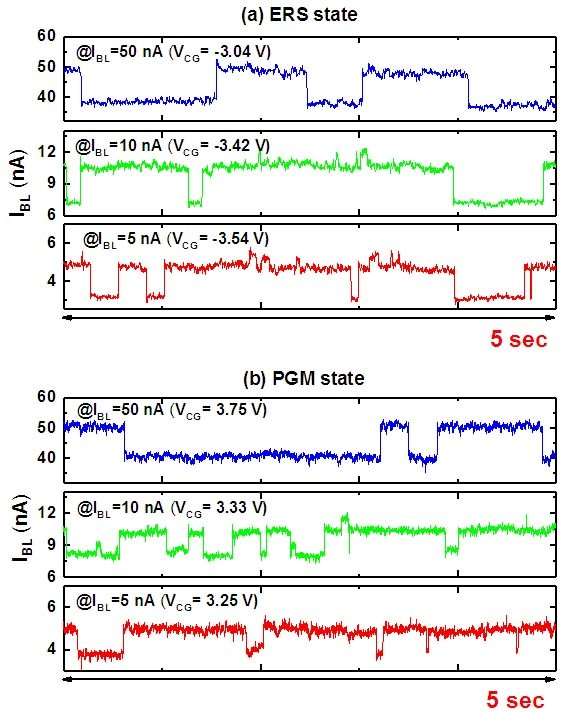However, as shown in Fig. 2, the extracted $\Delta I_ {BL}$ and $Delta I_ {BL}$/$I_ {BL}$ in the PGM state are less affected by the RTN property than those in the ERS state. In typical RTN characteristics, $Delta I_ {BL}$ increases as the $V _{CG}$ increases. On the other hand, the $Delta I_ {BL}$/$I_ {BL}$ is reduced because the number of current path increases at high $V _{CG} s$. The typical RTN properties with $V _{CG}$ change are observed in both states. The ERS and PGM states show appreciable difference when comparing the decrease of $Delta I_ {BL}$/$I_ {BL}$ with increasing $I_ {BL}$. The $Delta I_ {BL}$/$I_ {BL}$ decreased from 34% to 22% in the ERS state, while the $Delta I_ {BL}$/$I_ {BL}$ decreased from 23% to 19.5% in the PGM state. This means that the RTN caused by electron capture/emission by the same trap gives a relatively small $Delta I_ {BL}$ in the PGM state cell, which indicates smaller trap effect on $Delta I_ {BL}$ in the PGM state cell on the electron concentration in the channel.

Fig. 2. Extracted $Delta I_ {BL}$/$I_ {BL}$ with increasing $I_ {BL}$ (5, 10 and 50 nA) obtained from the RTN waveforms in Fig. 1. The same cell is measured in (a) ERS, (b) PGM states.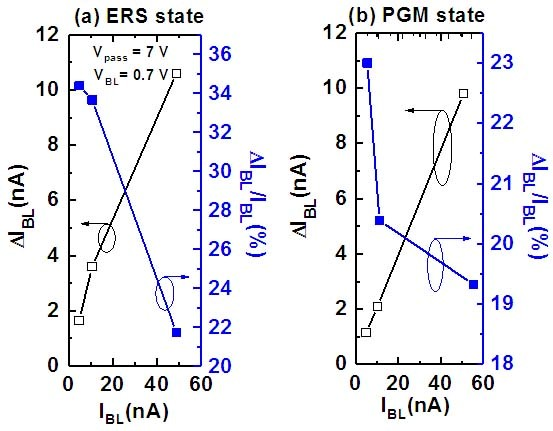To explain the cause of the RTN difference with different cell states, 3-D device simulation is performed. Fig. 3 shows the 3-D structure used in this simulation. As shown in the figure, simulated structure has gate-all-around structure with pillar oxide at the center of the cylinder. The length of WLs and the distance between adjacent WLs are 30 nm. The pillar oxide radius and the poly-Si channel thickness are 10 and 15 nm, respectively. The WLs are insulated from the channel by the gate insulator stack consists of a tunneling oxide / nitride / blocking oxide of 4/6/6 nm thickness. The nitride layer stores charges. To understand the different RTN properties between the ERS and PGM states, the simulation is performed with a hole and electron concentration of 1019 cm-3 stored in the nitride layer at each state. In order to increase the reliability of the simulation in this work, physical parameters such as trap density and carrier mobility calibrated with measurement data are used. The models used in this simulation are Shockley-Read-Hall (SRH) recombination and band-to-band generation models. In this simulation result, contours of electron concentration in the channel at two different $I_ {BL} s$ (1 and 10 nA) are observed in two cell states. Note that $I_ {BL}$ is different from that in the RTN measurement, but 1 nA and 10 nA correspond to low $V_ {CG}$ and high $V_ {CG}$. Here, high $V_ {CG}$ means a voltage slightly lower than $V_{th}$. Fig. 4(a) and Fig. 4(b) show the contour of the electron concentration in the channel of the cell in the ERS and PGM states, respectively. In the ERS state, electrons in the channel are distributed near the interface at both $I_ {BL} s$ as shown in Fig. 4(a). On the other hand, electrons in the channel in the PGM state at 1 nA of $I_ {BL}$ is distributed away from the interface due to repulsive force between channel electrons and electrons stored in the nitride layer as shown in Fig. 4(b). As a result, the electrons in the channel of the cell in the PGM state are distributed relatively far away from the interface where the trap is present compared to those in the ERS state, thereby further reducing noise. In particular, this effect is more clearly observed when the $I_ {BL}$ is low. Thus, it is explained that cells in the PGM state at low $I_ {BL}$ exhibit low noise characteristics.

Fig. 3. Schematic and cross sectional views of the 3-D NAND flash memory cell string structure used for 3-D TCAD simulation work.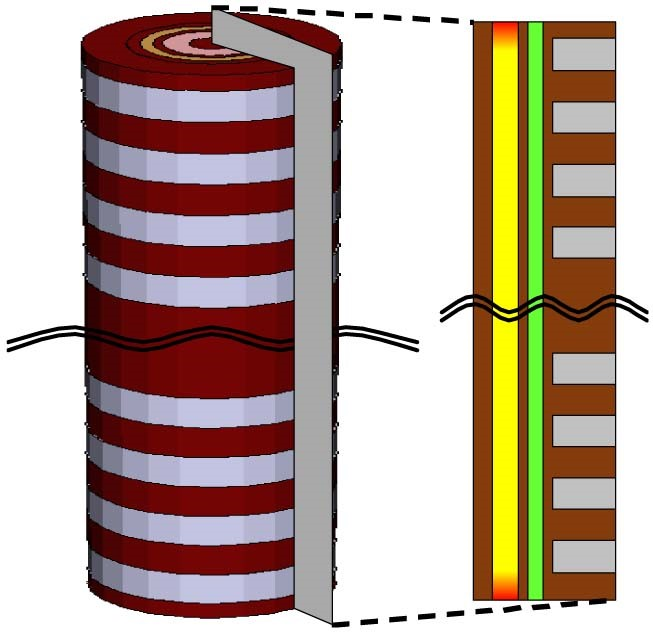Fig. 4. Simulated contours of the electron concentration in the channel at two different $I_ {BL} s$ ($I_ {BL}$ = 1 and 10 nA) in (a) the ERS, (b) the PGM states.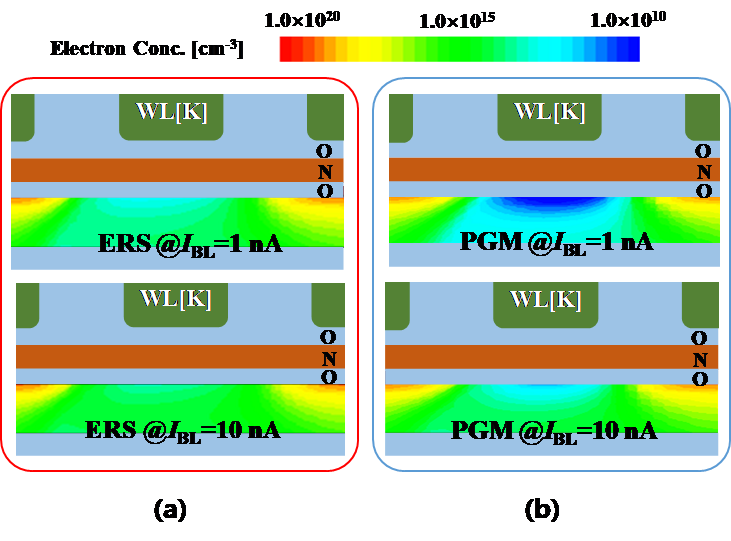To investigate LFN property difference in two cell states, noise properties of the cells in the PGM and ERS states are measured. To obtain the averaged LFN, the LFN is measured from the $I_ {BL}$ of WLs in the same position with multiple BLs as shown in Fig. 5(a). Fig. 5(b) shows the normalized noise power spectral density (PSD) of $I_ {BL}$ as a parameter of $I_ {BL}$. The noise PSDs of the ERS and PGM states are represented as solid and open symbols, respectively. When the $I_ {BL}$ is 100 µA (which means high $V_ {CG}$), the normalized noise PSDs of two cell states are quite similar and decrease with a 1/f slope with increasing frequency because the effects of multiple traps are superimposed under a high $V_ {CG}$. At high $V_ {CG}$, the electrons in the channel are distributed closer to the interface. In this case, the PSDs of both states reflect the trap characteristics of the interface. However, at $I_ {BL}$ less than 100 nA, the noise PSDs of two states are different. The noise PSDs of the ERS state is higher than those of PGM state as shown in Fig. 5(b). This phenomenon is clearly observed when the $I_ {BL}$ is 1 $\mu A$ and 100 $nA$, as indicated in the dashed ellipse. Small $I_ {BL}$ means low $V_ {CG}$, where the electrons in the channel are less affected by the interface trap of the devices.

Fig. 5. (a) Schematic circuit diagram used for LFN measurement with multi BLs connected in parallel, (b) Normalized noise power spectral density of the cells in the ERS and PGM states as a parameter of $I_ {BL}$.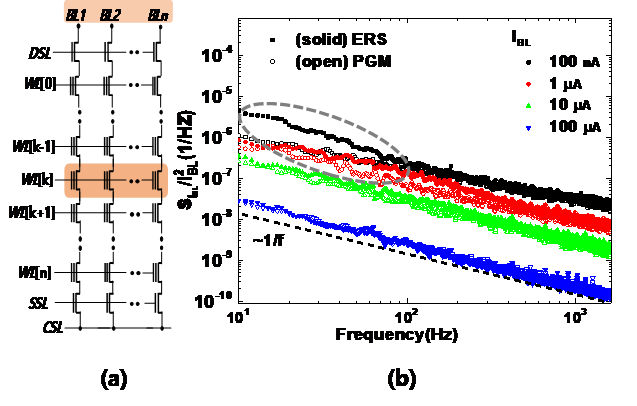## III. CONCLUSION

We have studied the RTN property in two different cell states of a cell in 3-D NAND flash memory cell string. At the same low $I_{BL}$, the $\Delta I_{BL}$ of the cell in the ERS state is larger since traps at the interface between tunneling oxide and poly-Si channel causing the RTN than those in the PGM state. The same result was also observed in low frequency noise characteristics. At low $I_{BL}$, the normalized noise PSD of the cell in the PGM state is smaller than that of the cell in the ERS state. The reason for these phenomena is that the electrons in the channel are distributed relatively far away from the interface between the poly-Si body and the tunneling oxide. In 3-D TCAD simulation, we have confirmed this electron distribution in the cell in the PGM state due to the repulsive force between the channel electrons and the electrons stored in the nitride layer at low $I_{BL}$.

### ACKNOWLEDGMENTS

This work was supported by BK21 program, KOREA.

### REFERENCES

1
Ishiduki M., et al , 2009, Optimal device structure for pipe-shaped BiCS Flash memory for ultra high density storage device with excellent performance and reliability, IEDM Tech. Dig., pp. 625-6282
Jang J., et al , 2009, Vertical cell array using TCAT (Terabit Cell Array Transistor) technology for ultra high density NAND flash memory, VLSI Tech. Dig., pp. 192-1933
Choi E., Park S. K., 2012, Device Considerations for High Density and Highly Reliable 3D NAND Flash Cell in Near Future, IEDM Tech. Dig., pp. 9.4.1-9.4.44
Tsai W. J., et al , 2016, Polycrystalline-silicon channel trap induced transient read instability in a 3D NAND flash cell string, IEDM Tech. Dig., pp. 11.3.1-11.3.45
Lun Z., et al , 2015, Investigation of the impact of grain boundary on threshold voltage of 3-D MLC NAND flash memory, IEEE Silicon Nanoelectronics Workshop, pp. 1-26
Hsiao Y. H., et al , 2014, Modeling the impact of random grain boundary traps on the electrical behavior of vertical gate 3-D NAND flash memory devices, IEEE Trans. Electron Devices, Vol. 61, No. 6, pp. 2064-20707
Wang P. Y., Tsui B. Y., 2015, A novel approach using discrete grain boundary traps to study the variability of 3-D vertical gate NAND flash memory cells, IEEE Trans. Electron Devices, Vol. 62, No. 8, pp. 2488-24938
Kang H. J., et al , 2014, Effect of Traps on Transient Bit-line Current Behavior in Word-line Stacked NAND Flash Memory with Poly-Si Body, VLSI Tech. Dig., pp. 28-299
Jeong M. K., et al , 2012, Characterization of traps in 3-D stacked NAND flash memory devices with tube-type poly-Si channel structure, IEDM Tech. Dig., pp. 9.3.1-9.3.410
Jeong M. K., et al , 2012, Analysis of Random Telegraph Noise and low frequency noise properties in 3-d stacked NAND flash memory with tube-type poly-Si channel structure, VLSI Tech. Dig., pp. 55-5611
Kang D., et al , 2014, A New Approach for Trap Analysis of Vertical NAND Flash Cell using RTN Characteristics, IEDM Tech. Dig., pp. 14.5.1-14.5.412
Lee et al S. K., et al , 2014, Investigation of Novel Inspection for 3D NAND Device Wordline Inspection, ASME 2014 25th anuual SEMI, pp. 27813
Joe S. M., et al , 2011, Threshold Voltage Fluctuation by Random Telegraph Noise in Floating Gate nand Flash Memory String, IEEE Trans. Electron Devices, Vol. 58, No. 1, pp. 67-7314
Joe S. M., et al , 2016, Diode-Type NAND Flash Memory Cell String Having Super-Steep Switching Slop Based on Positive Feedback, IEEE Trans. Electron Devices, Vol. 63, No. 4, pp. 1533-1538## Author

received the B.S. degree in electrical engineering from Seoul National University (SNU), Seoul, South Korea, in 2014, where he is currently pursuing the Ph.D. degree with the Department of Electrical and Computer Engineering.

received the Ph.D. degree in the Department of Electrical and Computer Engineering from Seoul National University (SNU), Seoul, in 2018, in.

He is currently working in SK-Hynix.

(F’16) received the Ph.D. degree from Seoul National University (SNU), Seoul, in 1993, in electronic engineering.

He was a Post-Doctoral Fellow with the Massachusetts Institute of Tech-nology, Cambridge, MA, USA, from 1998 to 1999.

He has been a Professor with the School of Electrical and Computer Engineering, SNU, since 2009.